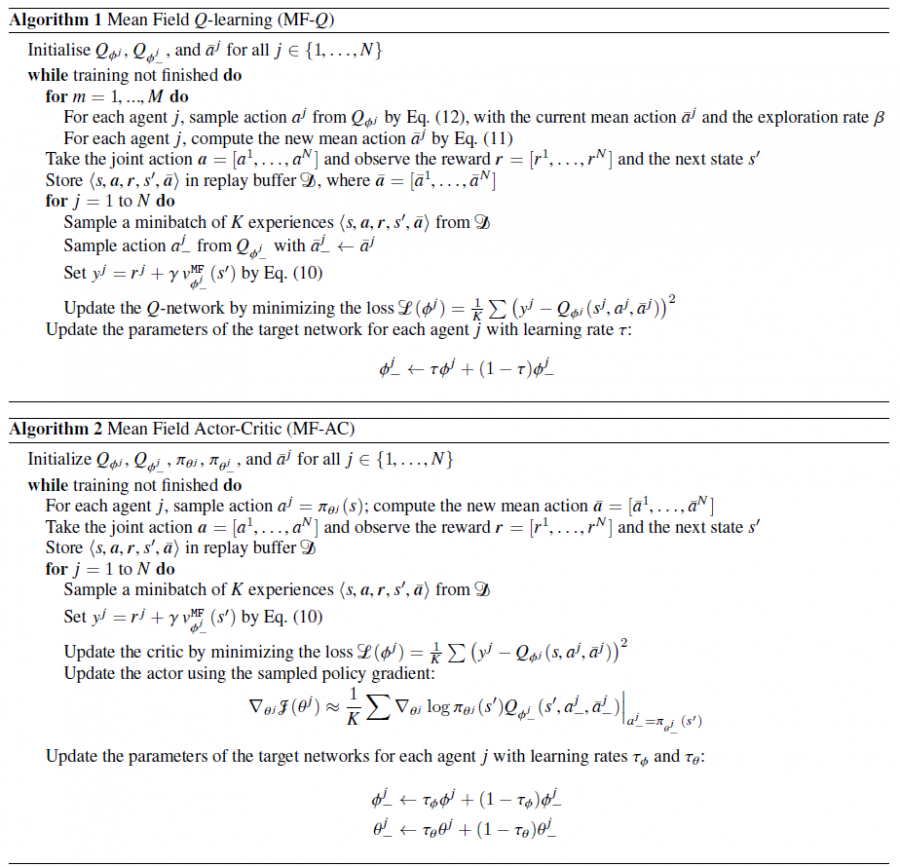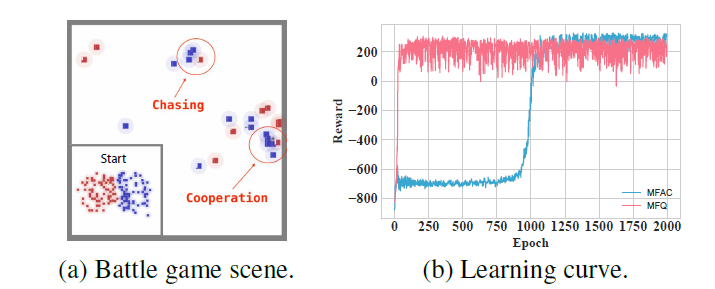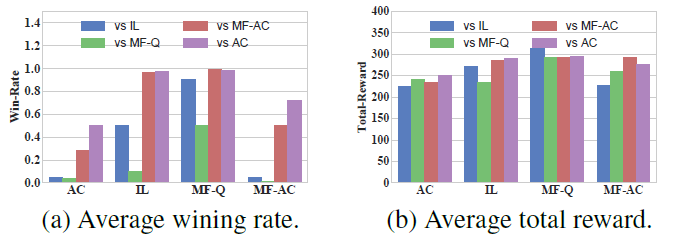# 多智能体强化学习算法

## Mean Field Multi-Agent Reinforcement Learning

Posted by MY on September 17, 2020

## 一、问题

MFMARL算法借用了平均场论（Mean Field Theory，MFT）的思想，其对多智能体系统给出了一个近似假设：对某个智能体，其他所有智能体对其产生的作用可以用一个均值替代。这样就就将一个智能体与其邻居智能体之间的相互作用简化为两个智能体之间的相互作用（该智能体与其所有邻居的均值）。这样极大地简化了智能体数量带来的模型空间的增大。应用平均场论后，学习在两个智能体之间是相互促进的：单个智能体的最优策略的学习是基于智能体群体的动态；同时，集体的动态也根据个体的策略进行更新。

## 二、解法

MFMARL算法主要解决的是集中式多智能体强化学习中，联合动作的维度随智能体数量n的增多极速扩大的情况。因为每个智能体是同时根据联合策略估计自身的值函数，因此当联合动作空间很大时，学习效率及学习效果非常差。为了解决这个问题，算法将值函数$Q^{j}(s, a)$转化为只包含邻居之间相互作用的形式： $Q^{j}(s, a)=\frac{1}{N^{j}} \sum_{k \in \mathcal{N}(j)} Q^{j}\left(s, a^{j}, a^{k}\right)$ 其中$N^{j}$表示智能体j邻居智能体的标签集， $N_{j}=|N(j)|$表示邻居节点的个数。上式对智能体之间的交互作用进行了一个近似，降低了表示智能体交互的复杂度，并且保留了部分主要的交互作用（近似保留邻居之间的交互，去掉了非邻居之间的交互）。虽然对联合动作$a$做了近似化简，但是状态信息$s$依然是一个全局信息。

#### 2.1 Mean Field 近似

$a_{k}=a_{j}+\delta a_{j, k}, \quad \text { where } \bar{a}_{j}=\frac{1}{N_{j}} \sum_{k} a_{k}$

\begin{array}{c} Q^{j}(s, a)=\frac{1}{N^{j}} \sum_{k} Q^{j}\left(s, a^{j}, a^{k}\right) \\ \begin{aligned} =\frac{1}{N^{j}} & \sum_{k}\left[Q^{j}\left(s, a^{j}, \bar{a}^{j}\right)+\nabla_{\bar{a}^{j}} Q^{j}\left(s, a^{j}, \bar{a}^{j}\right) \cdot \delta a^{j, k}\right.\\ &\left.+\frac{1}{2} \delta a^{j, k} \cdot \nabla_{\tilde{a}^{j, k}} Q^{j}\left(s, a^{j}, \tilde{a}^{j, k}\right) \cdot \delta a^{j, k}\right] \end{aligned} \\ =Q^{j}\left(s, a^{j}, \bar{a}^{j}\right)+\nabla_{\bar{a}^{j}} Q^{j}\left(s, a^{j}, \bar{a}^{j}\right) \cdot\left[\frac{1}{N^{j}} \sum_{k} \delta a^{j, k}\right] \\ +\frac{1}{2 N^{j}} \sum_{k}\left[\delta a^{j, k} \cdot \nabla_{\tilde{a}^{j}, k}^{2} Q^{j}\left(s, a^{j}, \tilde{a}^{j, k}\right) \cdot \delta a^{j, k}\right] \end{array}

$Q_{j, t+1}\left(s, a_{j}, \bar{a}_{j}\right)=(1-\alpha) Q_{j, t}\left(s, a_{j}, \bar{a}_{j}\right)+\alpha\left[r_{j}+\gamma v_{j, t}\left(s^{\prime}\right)\right]$

$v_{j, t}\left(s^{\prime}\right)=\sum_{a_{j}} \pi_{j, t}\left(a_{j} | s, \bar{a}_{j}\right) E_{\bar{a}_{j}\left(a_{-j} \sim \pi_{-j, t}\right)}\left[Q_{j, t}\left(s^{\prime}, a_{j}, \bar{a}_{j}\right)\right]$

$\bar{a}_{j}=\frac{1}{N_{j}} \sum_{k} a_{k}, \quad \text { where } a_{k} \sim \pi_{k, t}\left(\cdot | s, \bar{a}_{k-}\right)$

$\pi_{j, t}\left(a_{j} | s, \bar{a}_{j}\right)=\frac{\exp \left(-\beta Q_{j, t}\left(s, a_{j}, \bar{a}_{j}\right)\right)}{\sum_{a j \in A_{j}} \exp \left(-\beta Q_{j, t}\left(s, a_{j^{\prime}}, \bar{a}_{j}\right)\right)}$

$\mathcal{H}^{M F} Q(s, a)=E_{s^{\prime} \sim p}\left[r(s, a)+\gamma v^{M F}\left(s^{\prime}\right)\right]$

#### 2.2 算法设计## 三、实验内容## 四、缺点

MFMARL算法主要解决的是联合动作的维度随智能体数量增多的扩张问题，将$a$的维度缩减为$\left[a_{j}, \bar{a}_{j}\right]$。但是各个智能体的策略还是需要直到全局的状态信息$s$，不算是一个分布式的算法，并且依赖于通信获取邻居智能体的动作$a_{k}$。

## 五、优点

##### Share